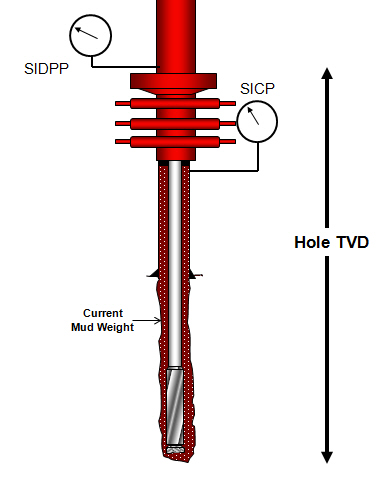# Formation Pressure from Kick Analysis

Once you shut in the well in and obtain shut in drill pipe pressure, you can estimate formation pressure by applying the hydrostatic pressure concept.

This following equation demonstrates you how to figure out formation pressure from the kick analysis.

Formation Pressure = SIDDP + (0.052 x Hole TVD x Current Mud Weight)Formation Pressure from Kick Analysis

Unit of each parameter is listed below:

Formation Pressure in psi

SIDDP (shut in drill pipe pressure) in psi

Hole TVD (true vertical depth) in ft

Current Mud Weight in ppg

Example: Well depth = 8,500’MD/8,000 TVD.

SIDPP = 300 psi

Current mud weight = 10.0 ppg

What is the formation pressure in psi?

Formation Pressure = 300+ (0.052 x 8000 x 10.0)

Formation pressure = 4,460 psi

Ref book:Formulas and Calculations for Drilling, Production and Workover, Second Edition

Share the joy